## Pythagoras's Theorem

Proves that the Diagonal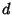of a Square with sides of integral length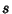cannot be Rational. Assume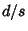is rational and equal to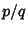where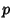and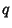are Integers with no common factors. Then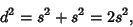so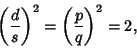and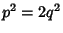, so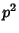is even. But ifis Even, thenis Even. Sinceis defined to be expressed in lowest terms,must be Odd; otherwiseandwould have the common factor 2. Sinceis Even, we can let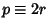, then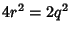. Therefore,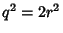, and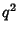, somust be Even. Butcannot be both Even and Odd, so there are noandsuch thatis Rational, andmust be Irrational.

In particular, Pythagoras's Constant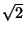is Irrational. Conway and Guy (1996) give a proof of this fact using paper folding, as well as similar proofs for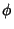(the Golden Ratio) and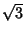using a Pentagon and Hexagon.

Pappas, T. Irrational Numbers & the Pythagoras Theorem.'' The Joy of Mathematics. San Carlos, CA: Wide World Publ./Tetra, pp. 98-99, 1989.